﻿ 基于自编码网络的导弹攻击区实时计算方法Download PDF文章快速检索 高级检索

Real-time calculation of missile launch envelope based on auto-encoder network
HU Dongyuan, YANG Rennong, YAN Mengda, YUE Longfei, ZUO Jialiang, WANG Ying
Air Traffic Control and Navigation College, Air Force Engineering University, Xi'an 710051, China
Abstract: Traditional three-line attack area of medium-range air-to-air missile can't provide effective tactical decision information for the pilot, and it has poor time effectiveness and low precision in calculation using fire control system. To address these problems, a new type of missile launch envelope, under the consideration of the target escape maneuver, is proposed with the attack aircraft as the center. In this paper, common and effective tactical maneuvers, which are used to escape from missiles successfully, are considered and analyzed through many classic examples of battles. The attack area is abstracted into seven killing envelops. the calculated methods are given and the offline simulations are carried out. The sample database is established by identifying eight motion parameters and envelop-values. By introducing deep learning methods, the Auto-Encoder (AE) network model is constructed. By adopting unsupervised learning, the primary features of the sample are extracted, and the high dimensional vectors describing nonlinearity in the sample database are obtained. The deep network model is established with high dimensional vectors to extract advanced features for fitting. Experimental results show that the loss of the deep network can be controlled within 15 m compared with the six-degree-of-freedom simulation results and the actual missile data. The online calculation time is only 0.04 s, which can meet the real-time requirements. The new missile envelope can provide timely and effective information for pilot to understand enemy situation and support maneuvering decision-making.
Keywords: air to air missile    attack area    killing envelop    deep learning    auto-encoder network    real time

1) 攻击区发射包线的仿真计算。该类方法根据运动学、飞行力学进行仿真计算，如三自由度[7-8]和六自由度[9-10]导弹仿真模型。三自由度模型虽较六自由度有所简化，但都需要较长计算时间，无法用于火控系统的在线解算。

2) 攻击区快速拟合。主要有多项式拟合[11-12]、最小二乘法拟合和神经网络法[14-16]。在计算速度上有所提高，但计算结果误差较大。

3) 查表法和插值法方面。查表法是将影响攻击区的各关键参数与包线距离值制成表格，利用表格中的数据线性插值近似替代所求值。这种方法在火控系统中需要占用大量的内存，并且精度损失非常严重。

1 新型杀伤包线数学模型 1.1 导弹攻击区分析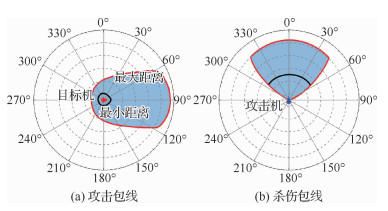图 1 空空导弹攻击区示意图 Fig. 1 Schematic of air-to-air missile envelop

1.2 新型杀伤包线概念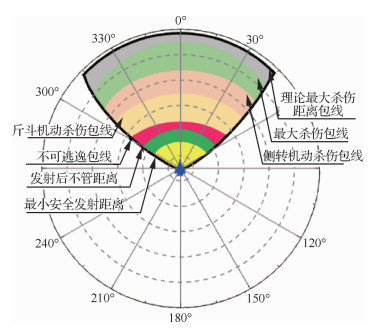图 2 7条新型杀伤包线示意图 Fig. 2 Schematics of seven missile killing envelops

1) 理论最大杀伤距离包线

2) 最大杀伤包线

3) 侧转机动杀伤包线

4) 斤斗机动杀伤包线

5) 不可逃逸包线

6) 发射后不管距离

7) 最小安全发射距离

7条杀伤包线根据预测目标机可能实行的逃逸机动，将传统的最小距离和目标机之间的空域进行了详细划分，辅助飞行员掌握可能的攻击效果。根据7条杀伤包线定义建立数学模型，仿真求解不同敌我状态下的7条包线的精确值。

2 空空导弹弹道仿真模型

2.1 导弹运动模型

2.2 目标典型机动分析及运动仿真 2.2.1 目标典型机动类型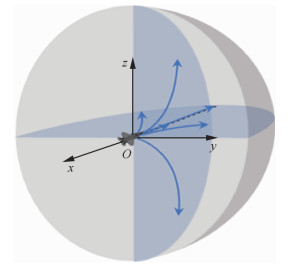图 3 基本操纵机动示意图 Fig. 3 Schematic of basic maneuver of pilot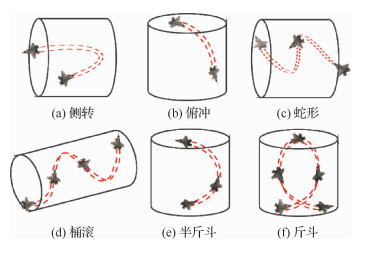图 4 战术机动示意图 Fig. 4 Schematic of tactical maneuver of pilot

6种机动格斗动作的组合可构成了双方交战中摆脱对方跟踪、规避导弹和抢占最佳方位的全过程。结合飞行训练系统(ACMI)中记录的数据对空战过程进行复盘还原，分析可知规避导弹主要有4种典型机动动作，分别为侧转、蛇形、俯冲和斤斗机动，数据还原如图 5所示。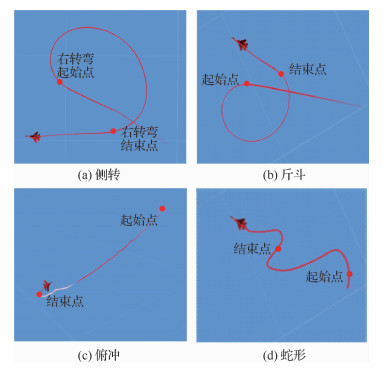图 5 空战机动轨迹 Fig. 5 Trajectory of air combat maneuver

2.2.2 目标运动模型方程

 $\left\{\begin{array}{l} \frac{\mathrm{d} x}{\mathrm{d} t}=V \cos \theta \cos \psi v \\ \frac{\mathrm{d} y}{\mathrm{d} t}=V \sin \theta \\ \frac{\mathrm{d} z}{\mathrm{d} t}=-V \cos \theta \sin \psi v \end{array}\right.$ （1）

 $\left\{\begin{array}{l} \frac{\mathrm{d} \vartheta}{\mathrm{d} t}=\omega_{y 1} \sin \gamma+\omega_{z 1} \cos \gamma \\ \frac{\mathrm{d} \psi}{\mathrm{d} t}=\frac{1}{\cos \vartheta}\left(\omega_{y 1} \cos \gamma-\omega_{z 1} \sin \gamma\right) \\ \frac{\mathrm{d} \gamma}{\mathrm{d} t}=\omega_{x 1}-\left(\omega_{y 1} \cos \gamma-\omega_{z 1} \sin \gamma\right) \tan \vartheta \end{array}\right.$ （2）
 $\left\{\begin{array}{l} m \frac{\mathrm{d} V}{\mathrm{d} t}=P \cos \alpha \cos \beta-X-m g \sin \theta \\ m V \frac{\mathrm{d} \theta}{\mathrm{d} t}=P\left(\sin \alpha \cos \gamma_{v}+\cos \alpha \cos \beta \sin \gamma_{V}\right)+ \\ \;\;\;\;Y\left(\cos \gamma_{v}-Z \sin \gamma_{v}-m g \cos \theta\right) \\ -m V \cos \theta \frac{\mathrm{d} \psi v}{\mathrm{d} t}=P\left(\sin \alpha \sin \gamma_{v}-\right. \\ \;\;\;\;\left.\cos \alpha \sin \beta \cos \gamma_{v}\right)+Y \sin \gamma_{v}+Z \cos \gamma_{v} \end{array}\right.$ （3）

 $\left\{\begin{array}{l} M_{x 1}=J_{x 1} \frac{\mathrm{d} \omega_{x 1}}{\mathrm{d} t}+\left(J_{z 1}-J_{y 1}\right) \omega_{z 1} \omega_{y 1} \\ M_{y 1}=J_{y 1} \frac{\mathrm{d} \omega_{y 1}}{\mathrm{d} t}+\left(J_{x 1}-J_{z 1}\right) \omega_{x 1} \omega_{z 1} \\ M_{z 1}=J_{z 1} \frac{\mathrm{d} \omega_{z 1}}{\mathrm{d} t}+\left(J_{y 1}-J_{x 1}\right) \omega_{y 1} \omega_{x 1} \end{array}\right.$ （4）

2.2.3 典型机动动作仿真复现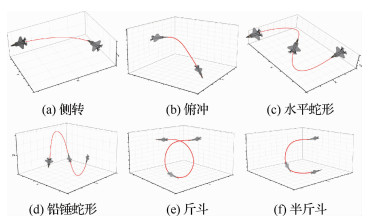图 6 机动仿真轨迹 Fig. 6 Simulation of maneuvering trajectories
2.3 弹目相对运动和导引规律

 $\frac{\mathrm{d} \sigma}{\mathrm{d} t}=K \frac{\mathrm{d} q}{\mathrm{d} t}$ （5）

3 离线仿真及分析 3.1 仿真限制条件

1) 距离限制

2) 时间限制

3) 速度限制

3.2 仿真流程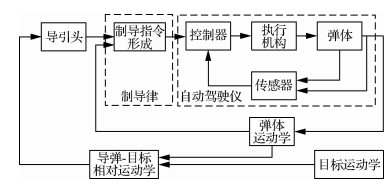图 7 导弹制导控制回路 Fig. 7 Circuit of missile guidance and control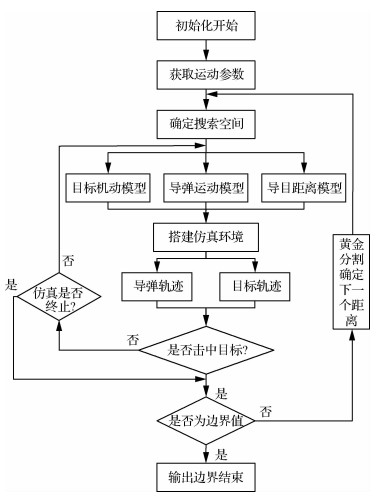图 8 杀伤包线计算流程 Fig. 8 Calculation process of missile killing envelop

3.3 仿真结果分析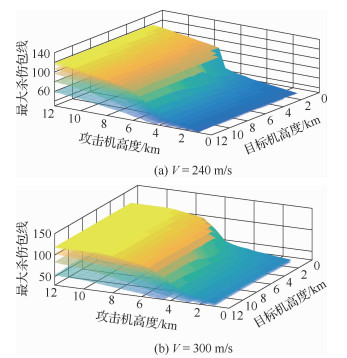图 9 不同高度下最大杀伤包线 Fig. 9 Max killing envelop at different altitudes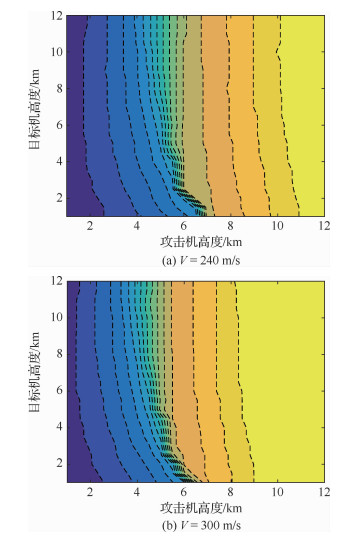图 10 不同高度变化对最大杀伤包线的影响 Fig. 10 Effect on max killing enrelop with changing altitudes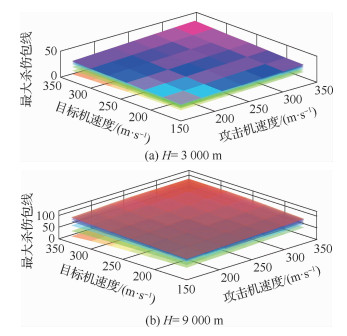图 11 不同速度下最大杀伤包线 Fig. 11 Max killing envelop at different speeds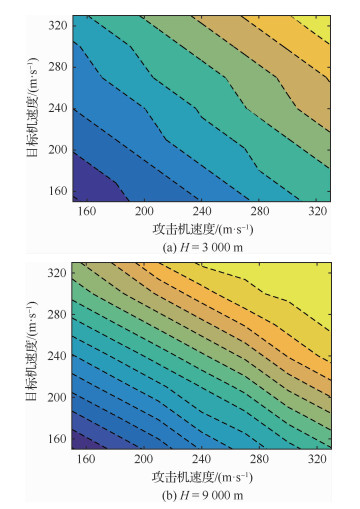图 12 不同速度变化对包线的影响 Fig. 12 Effect on milling envelop with changing speeds
4 基于自编码网络攻击区拟合 4.1 构建攻击包线样本库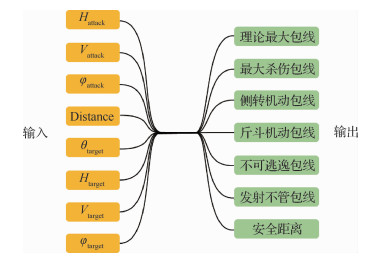图 13 杀伤包线样本库 Fig. 13 Database of killing envelop

1) 数据离散化

2) 训练集和测试集

3) 数据归一化

 $x^{*}=\frac{x-x_{\min }}{x_{\max }-x_{\min }}$
4.2 自编码网络模型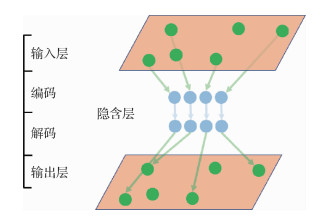图 14 简单自编码网络 Fig. 14 Single auto-encoder network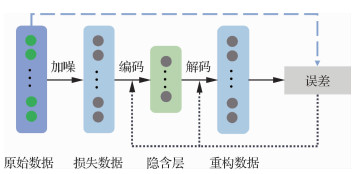图 15 降噪自编码网络 Fig. 15 Denoising auto-encoder network

4.3 模型构建及参数训练 4.3.1 模型构建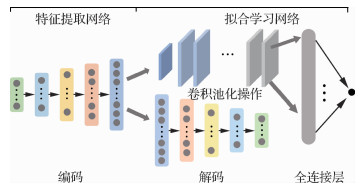图 16 深度学习拟合网络 Fig. 16 Deep learning network for fitting
4.3.2 网络深度设定

1) 随着网络深度增加，对样本中隐含规律的学习能力越强，网络提取抽象特征性能越好。

2) 增加隐含层数可以在一定程度上降低网络误差，提高精度，但隐含层越多，网络越复杂，增加了网络的训练时间。

3) 过拟合和梯度消失现象与网络隐层节点数量关系密切，节点越多，过拟合现象越明显。

4.3.3 网络参数设置

1) 高斯噪声

 $P(t)=\frac{1}{\sqrt{2 \mathsf{π}} \sigma} \exp \left(-\frac{(t-\mu)^{2}}{2 \sigma^{2}}\right)$

 $\mathrm{ERROR}=\frac{1}{n} \sum(y-\tilde{x})^{2}$

2) 学习率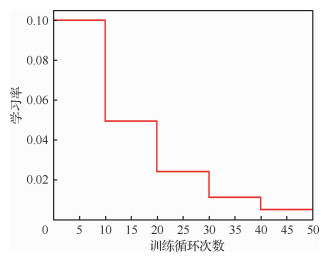图 17 网络训练中学习率的变化情况 Fig. 17 Variation of learning rate in network training

3) 初始权值

4) 权值粗调和微调

4.4 实验结果与分析 4.4.1 特征提取网络实验结果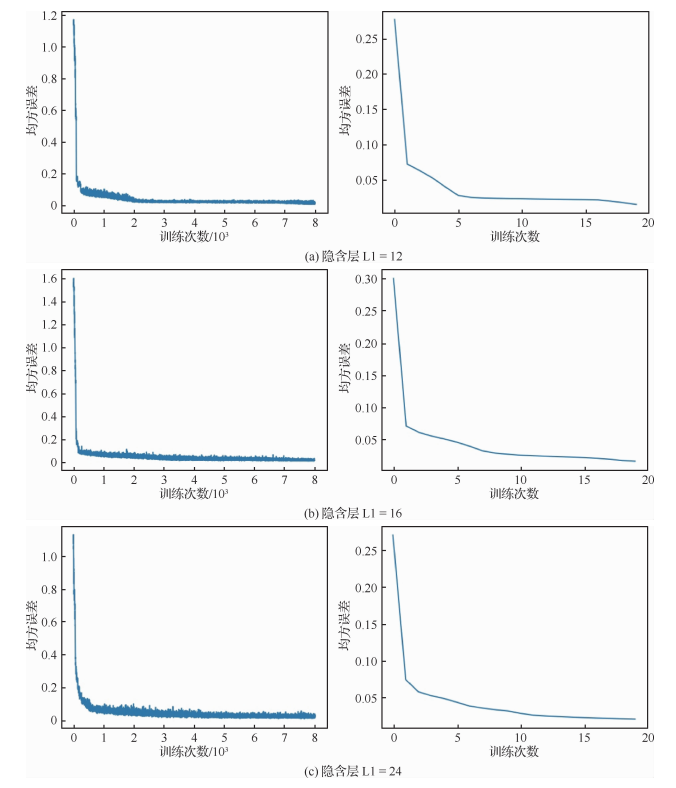图 18 隐含层节点与训练误差的关系 Fig. 18 Variation of training loss with different hidden layers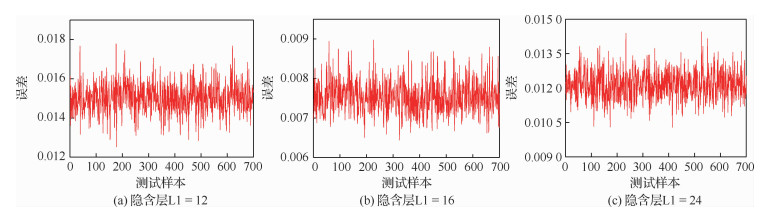图 19 隐含层节点与测试误差的关系 Fig. 19 Variation of test error with different hidden layers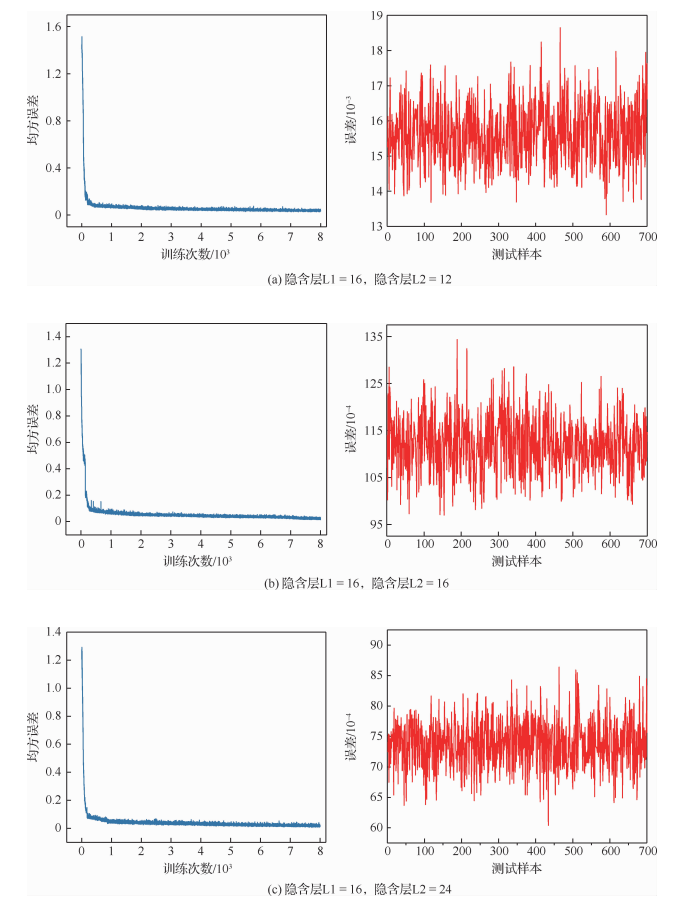图 20 两个隐含层与测试误差的关系 Fig. 20 Variation of test error with two different hidden layers图 21 3个隐含层与测试误差的关系 Fig. 21 Variation of test error with three different hidden layers

4.4.2 拟合学习网络实验结果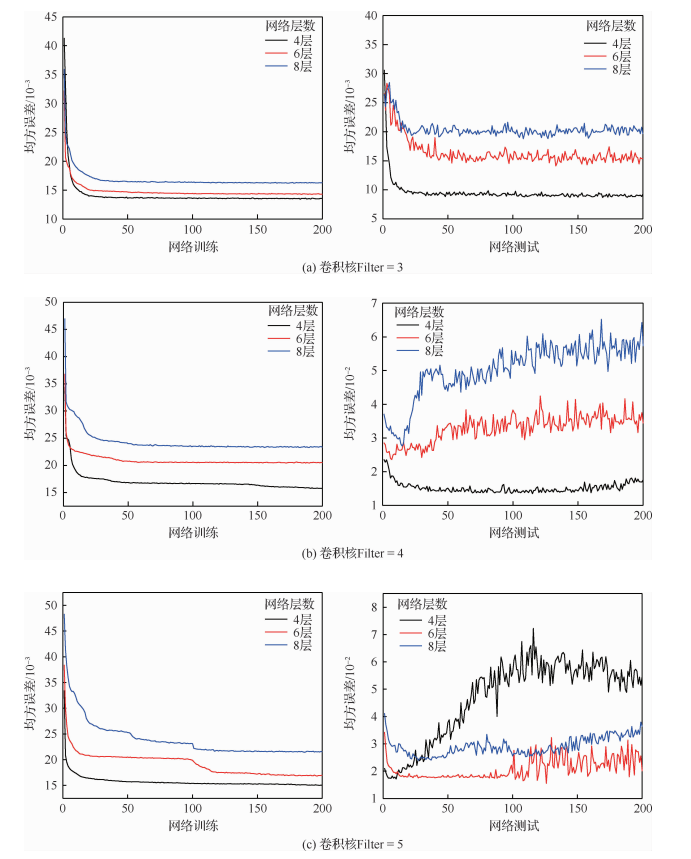图 22 卷积核和网络深度与测试误差的关系 Fig. 22 Variation of filter and depth of network with test error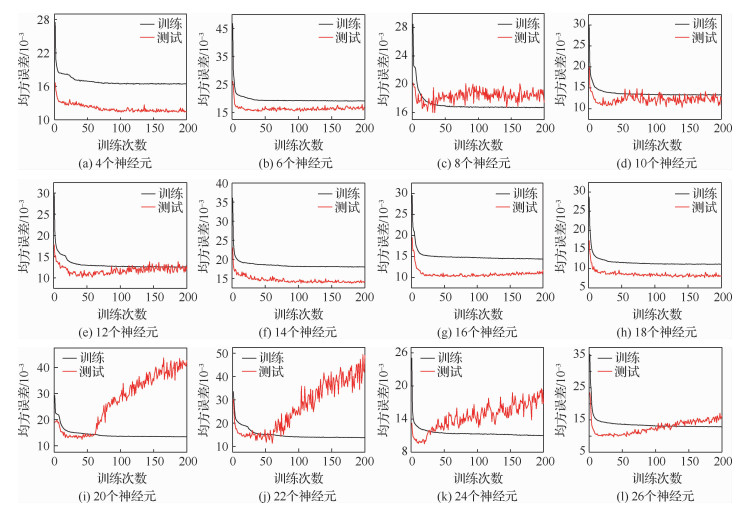图 23 全连接层节点数与训练误差的关系 Fig. 23 Variation of training error with different neurons of full connection layer
4.4.3 拟合结果及实效性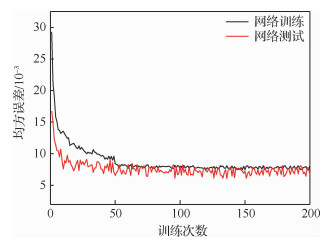图 24 整体网络训练误差 Fig. 24 Training error in completed network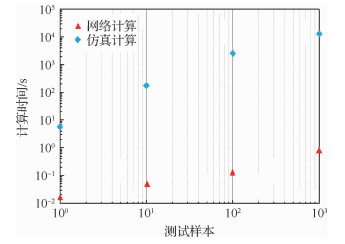图 25 深度网络计算时间
5 结论

1) 杀伤包线对发射机高度变化较为敏感，当发射机高度达到7 000 m以上时，高度不再是决定性因素，随着两机的高度的增加，影响程度减弱。

2) 杀伤包线考虑到目标的机动类型，为飞行员根据导弹攻击区把握战场态势提供了新方法，可以有效避免三线攻击区带来的态势漏洞。

3) 利用深度学习模型解算杀伤包线能够有效降低误差，提高计算精度，解算所需的时间能够满足空战实时性需求。

  黄睿, 刘小方, 郑祥. 某型导弹性能质量评估系统设计与实现[J]. 火炮发射与控制学报, 2019, 40(2): 88-92. HUANG R, LIU X F, ZHENG X. Design and implementation of a missile performance quality evaluation system[J]. Journal of Gun Launch & Control, 2019, 40(2): 88-92. (in Chinese) Cited By in Cnki | Click to display the text  史振庆, 梁晓龙, 张佳强, 等. 基于导弹攻击区的空战态势评估[J]. 火力与指挥控制, 2018, 43(9): 89-93. SHI Z Q, LIANG X L, ZHANG J Q, et al. Situation assessment for air combat based on missile attack zone[J]. Fire Control & Command Control, 2018, 43(9): 89-93. (in Chinese) Cited By in Cnki | Click to display the text  付昭旺, 于雷, 李战武, 等. 战斗机隐蔽接敌轨迹优化方法[J]. 国防科技大学学报, 2013, 35(5): 52-58. FU Z W, YU L, LI Z W, et al. The trajectory optimization method for fighter stealthy approach[J]. Journal of National University of Defense Technology, 2013, 35(5): 52-58. (in Chinese) Cited By in Cnki (3) | Click to display the text  董海霞, 邹杰, 王永庭. 基于协同攻击区模型的多机最优攻击占位决策[J]. 火力与指挥控制, 2018, 43(12): 11-15. DONG H X, ZOU J, WANG Y T. Collaborative attack area model for multi-aircrafts of optimal attack occupancy decision[J]. Fire Control & Command Control, 2018, 43(12): 11-15. (in Chinese) Cited By in Cnki | Click to display the text  WANG H, LEI H, YE J, et al. A novel capture region of retro proportional navigation guidance law for intercepting high-speed nonmaneuvering targets[J]. Proceedings of the Institution of Mechanical Engineers, Part G:Journal of Aerospace Engineering, 2018, 232(6): 86-98. Click to display the text  方学毅, 刘俊贤, 周德云. 基于背景插值的空空导弹攻击区在线模拟方法[J]. 系统工程与电子技术, 2019, 41(6): 1286-1293. FANG X Y, LIU J X, ZHOU D Y. Back ground interpolation for on-line simulation of capture zone of air-to-air missiles[J]. Systems Engineering and Electronics, 2019, 41(6): 1286-1293. (in Chinese) Cited By in Cnki | Click to display the text  周须峰, 易华, 谢永强. 近距格斗空空导弹三自由度弹道仿真建模[J]. 四川兵工学报, 2014, 35(2): 9-12. ZHOU X F, YI H, XIE Y Q. Modeling on 3DOF trajectory simulation of short range dogfight air-to-air missile[J]. Journal of Sichuan Ordnance, 2014, 35(2): 9-12. (in Chinese) Cited By in Cnki | Click to display the text  黄威, 任洋. 基于自适应步长的空空导弹攻击区解算方法[J]. 电光与控制, 2019, 26(5): 55-58. HUANG W, REN Y. Adaptive step-size based calculation of air-to-air missile launch envelopes[J]. Electronics Optics & Control, 2019, 26(5): 55-58. (in Chinese) Cited By in Cnki | Click to display the text  高胜灵, 胡松启. 基于Matlab/Simulink的导弹六自由度弹道仿真系统设计[J]. 科学技术与工程, 2011, 11(1): 29-34. GAO S L, HU S Q. Six-degree-of-freedom simulation modelling for missile trajectory based on Matlab/Simulink[J]. Science Technology and Engineering, 2011, 11(1): 29-34. (in Chinese) Cited By in Cnki (18) | Click to display the text  程翔, 李苑青, 王丽华. 基于OpenGL的六自由度三维弹道仿真技术研究[J]. 电子科技, 2017, 30(6): 16-20. CHENG X, LI Y Q, WANG L H. Research on six degree of freedom 3d trajectory simulation based on opengl[J]. Electronic Science and Technology, 2017, 30(6): 16-20. (in Chinese) Cited By in Cnki | Click to display the text  俞吉, 周德云, 周灿辉. 基于云模型空空导弹攻击区的拟合方法[J]. 火力与指挥控制, 2013, 38(7): 32-35. YU J, ZHOU D Y, ZHOU C H. A method for approximating allowable attack area for air-to-air missile based on cloud model[J]. Fire Control & Command Control, 2013, 38(7): 32-35. (in Chinese) Cited By in Cnki (2) | Click to display the text  邓健, 王星, 曾艳丽, 等. 基于数据建模的空空导弹攻击区仿真[J]. 弹箭与制导学报, 2016, 36(4): 33-35. DENG J, WANG X, ZENG Y L, et al. Simulation of attack area for air-to-air missile based on data modeling[J]. Journal of Projectiles, Rockets, Missiles and Guidance, 2016, 36(4): 33-35. (in Chinese) Cited By in Cnki | Click to display the text  张列航, 雷蕾, 杨长启. 中程空空导弹攻击区仿真与拟合[J]. 航空科学技术, 2010(4): 25-27. ZHANG L H, LEI L, YANG C Q. Attack area calculating and data fitting for medium air-to-air missile[J]. Aeronautical Science & Technology, 2010(4): 25-27. (in Chinese) Cited By in Cnki (4) | Click to display the text  史振庆, 梁晓龙, 张佳强, 等. 基于GWO-BP神经网络的攻击区解算方法[J]. 飞行力学, 2019, 37(3): 64-67, 92. SHI Z Q, LIANG X L, ZHANG J Q, et al. Solution of attack zone based on GWO-BP neural network[J]. Flight Dynamics, 2019, 37(3): 64-67, 92. (in Chinese) Cited By in Cnki | Click to display the text  孟博. 基于BP神经网络的空空导弹攻击大机动目标攻击区仿真研究[J]. 弹箭与制导学报, 2017, 37(4): 43-46, 50. MENG B. Research on launch envelopes simulation of air-to-air missile attacking high maneuvering targets based on bp neural network[J]. Journal of Projectiles, Rockets, Missiles and Guidance, 2017, 37(4): 43-46, 50. (in Chinese) Cited By in Cnki | Click to display the text  苗涛, 杨毅, 南英. 导弹动态可攻击区实时在线算法研究[J]. 飞行力学, 2018, 36(2): 39-43. MIAO T, YANG Y, NAN Y. Research on real-time online algorithm of missile dynamic attack zone[J]. Flight Dynamics, 2018, 36(2): 39-43. (in Chinese) Cited By in Cnki | Click to display the text  王志刚, 张宁, 李伟. 一种空空导弹攻击区的快速解算方法[J]. 固体火箭技术, 2014, 37(4): 448-452. WANG Z G, ZHANG N, LI W. A fast solving method for attacking area of air-to-air missile[J]. Journal of Solid Rocket Technology, 2014, 37(4): 448-452. (in Chinese) Cited By in Cnki (5) | Click to display the text  李枭扬, 周德云, 冯琦, 等. 基于遗传规划的空空导弹攻击区拟合[J]. 弹箭与制导学报, 2015, 35(3): 16-18, 22. LI X Y, ZHOU D Y, FENG Q, et al. Air-to-air missile launch envelops fitting based on genetic programming[J]. Journal of Projectiles, Rockets, Missiles and Guidance, 2015, 35(3): 16-18, 22. (in Chinese) Cited By in Cnki (1) | Click to display the text  徐国训, 梁晓龙, 张佳强, 等. 双机空空导弹协同攻击区仿真研究[J]. 火力与指挥控制, 2019, 44(1): 34-39. XU G X, LIANG X L, ZHANG J Q. Simulation research on air-to-air missile cooperating weapon engagement zone of two aircrafts[J]. Fire Control & Command Control, 2019, 44(1): 34-39. (in Chinese) Cited By in Cnki | Click to display the text  高劲松, 赵华超, 田省民. 空空导弹的两种全向攻击方式的关系[J]. 电光与控制, 2018, 25(12): 16-20. GAO J S, ZHAO H C, TIAN X M. On relationship between two modes of aam's all-aspect attack[J]. Electronics Optics & Control, 2018, 25(12): 16-20. (in Chinese) Cited By in Cnki | Click to display the text  左家亮, 杨任农, 张滢, 等. 分布式半实物环境实时弹道仿真步长自适应研究[J]. 兵工学报, 2015, 36(4): 653-659. ZUO J L, YANG R N, ZHANG Y, et al. Research on the step size of real-time self-adaptation trajector[J]. Acta Armamentar, 2015, 36(4): 653-659. (in Chinese) Cited By in Cnki | Click to display the text  张彬超, 寇雅楠, 邬蒙, 等. 基于深度置信网络的近距空战态势评估[J]. 北京航空航天大学学报, 2017, 43(7): 1450-1459. ZHANG B C, KOU Y N, WU M, et al. Close-range air combat situation assessment using deep belief network[J]. Journal of Beijing University of Aeronautics and Astronautics, 2017, 43(7): 1450-1459. (in Chinese) Cited By in Cnki (4) | Click to display the text  焦珂, 皮亦鸣, 陆继珍. 空空导弹发射包线的快速计算方法[J]. 火力与指挥控制, 2010, 35(6): 100-102, 106. JIAO K, PI Y M, LU J Z. A fast calculation method of launch envelope of air-to-air missile[J]. Fire Control & Command Control, 2010, 35(6): 100-102, 106. (in Chinese) Cited By in Cnki (4) | Click to display the text  刘瑛, 李敏强, 张瑞峰. 复杂机动动作最优航迹控制模型及操纵特性分析[J]. 控制理论与应用, 2014, 31(5): 566-576. LIU Y, LI M Q, ZHANG R F. The optimal trajectory control model of the aircraft maneuver and its operation characteristics[J]. Control Theory & Applications, 2014, 31(5): 566-576. (in Chinese) Cited By in Cnki (6) | Click to display the text  HUI Y, NAN Y, CHEN S, et al. Dynamic attack zone of air-to-air missile after being launched in random wind field[J]. Chinese Journal of Aeronautics, 2015, 28(5): 1519-1528. Click to display the text  FANG J, ZHANG L, WEI F, et al. Approximate dynamic programming for CGF air combat maneuvering decision[C]//Proceedings of the 20162nd IEEE International Conference on Computer and Communications (ICCC). Piscataway, NJ: IEEE Press, 2016.  贾镇泽, 樊晓光, 薛明浩, 等. 基于机动动作元的敌机战术机动在线识别方法[J]. 北京理工大学学报, 2018, 38(8): 820-827. JIA Z Z, FAN X G, XUE M H, et al. Online identification method for tactical maneuver of target based on air combat maneuver element[J]. Transactions of Beijing Institute of Technology, 2018, 38(8): 820-827. (in Chinese) Cited By in Cnki | Click to display the text  FANG J, ZHANG L, WEI F, et al. Approximate dynamic programming for CGF air combat maneuvering decision[C]//IEEE International Conference on Computer & Communications. Piscataway, NJ: IEEE Press, 2017. Click to display the text  杨新, 王小虎, 申功璋, 等. 飞机六自由度模型及仿真研究[J]. 系统仿真学报, 2000(3): 36-39. YANG X, WANG X H, SHEN G Z, et al. Modeling and simulation research of six-degree-of-freedom fighter[J]. Journal of System Simulation, 2000(3): 36-39. (in Chinese) Cited By in Cnki (153) | Click to display the text  方振平. 航空飞行器飞行动力学[M]. 北京: 北京航空航天大学出版社, 2005. FANG Z P. Aviation vehicle flight dynamics[M]. Beijing: Beihang Universtity Press, 2005. (in Chinese)  董肖杰, 余敏建, 宋帅.空战机动动作库及控制算法设计研究[C]//第五届中国指挥控制大会, 2017. DONG X J, YU M J, SONG S. Research on the design of air combat maneuver library and control arithmetic of movements[C]//Proceedings of the 5th China Command and Control Conference, 2017(in Chinese).  林德福, 王辉, 王江, 等. 战术导弹自动驾驶仪设计与制导律分析[M]. 北京: 北京理工大学出版社, 2012. LIN D F, WANG H, WANG J, et al. Autopilot design and guidance law analysis for tactical missile[M]. Beijing: Beijing Institute of Technology Press, 2012. (in Chinese)  潘广源, 柴伟, 乔俊飞. DBN网络的深度确定方法[J]. 控制与决策, 2015, 30(2): 256-260. PAN G Y, CHAI W, QIAO J F. Calculation for depth of deep belief network[J]. Control and Decision, 2015, 30(2): 256-260. (in Chinese) Cited By in Cnki (35) | Click to display the text
http://dx.doi.org/10.7527/S1000-6893.2019.23571

0

#### 文章信息

HU Dongyuan, YANG Rennong, YAN Mengda, YUE Longfei, ZUO Jialiang, WANG Ying

Real-time calculation of missile launch envelope based on auto-encoder network

Acta Aeronautica et Astronautica Sinica, 2020, 41(4): 323571.
http://dx.doi.org/10.7527/S1000-6893.2019.23571